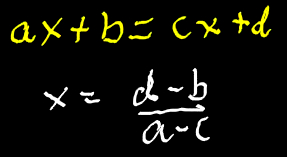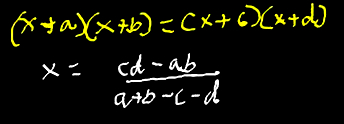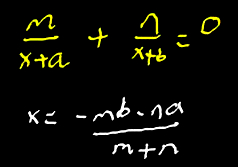# Vedic Math-1 Dubai UAE

Vedic Math-1 Algebra Equations

## Basic Equations:

### Type 1:Example:

5x+2 = 3x + 6

=> x = (6-2)/(5-3) = 4/2 = 2

### Type 2:Example:

(x+1)(x+2)= (x+3)(x+4)

=> x= (12-2)/(1+2-3-4) = 10/(-4) = -5/2

### Type 3:Example:

(3x+2)/(2x+1) = 4/3

x = (4-6)/(9-8) = -2/1 = -2

### Type 4:Example:

1/(x+2) + 2/(x+1) = 0

=> x = (-1-4)/3 = -5/3

### First Method:

A term which occurs as a common factor in all the terms is equated to 0.

Examples:
12x+3x = 4x + 3x … As x is common factor both sides.So, x = 0.
9(x+3) = 4(x+3) … As (x+3) is common term both sides. So, x+3 = 0. x = -3.

### Second Method:

The product of the independent terms is same both sides then equated to 0.

Examples:
(x+5)(x+4) = (x+2)(x+10)
As product of independent terms (non-x terms): 5 x 4 = 2 x 10 , is same on both sides.
Therefore, x=0.

### Third Method:

The sum of the Denominators of two fractions having the same numerical numerator is equated to 0.

Examples:
1/(2x-1) + 1/(4x-1) = 0
Therefore, sum of denominators: 2x-1 + 4x-1 = 0
On solving x = 1/3

### Fourth Method:

The sum of the Numerators and the sum of the Denominators is the same, then that sum equated to 0.

Examples:
(2x +9)/ (2x +7) = (2x +7)/ (2x +9)
Thus, 2x + 9 + 2x + 7 = 0
Hence 4x + 16 = 0 hence x = -4

If there is a numerical factor in the algebraic sum, then we remove that factor
(3x +4)/ (6x +7) = (x +1)/ (2x +3)
Here, Addition of both numerators = 4x +5
Addition of both Denominators = 8x + 10 =2(4x +5)
where, 2 is the numerical factor. So remove it
4x +5 =0. Hence x= -5/4

NOTE:
In above both examples, when we do cross multiplication the x2 term is getting cancelled. So instead of being quadratic eq and having 2 roots, it becomes simple linear equation and THUS has single root.
But if x2 term is not getting cancelled then it becomes quadratic equation and thus will have 2 roots/values. See next Meaning.

### Fifth Method:

Same Meaning as that of Type 4

Examples:
(3x +4)/ (6x +7) = (5x +6)/ (2x +3)
Here as well like previous meaning, Addition of both numerators = Addition of both Denominators. So x = -5/4

BUT on cross multiplication x2 term is not getting cancelled so it is quadratic equation and thus will have to find the 2nd root as well,
Calculated as |D1 – D2| = |N1 – N2| = 2x + 2 = 0(On removal of numerical factor)
So x = -1.
So Values of x are -5/4 and -1.

### ax + by = p cx + dy = q

Solving,
x = (bq – pd) / (bc – ad)
y = (cp – aq) / (bc – ad)

Notice that for calculation of numerators (x any y) cyclic method is used and Denominators remains same for both x and y.

Examples:
2x + 3y =6
3x + 4y = 3

Applying above formula:
x = (9 – 24)/ (9 – 8) = -15
y = (18 – 6) (9 – 8) = 12

-3x + 5y = 2
4x + 3y = -5

Applying above formula:
x = (-25 -6) / (20+9) = -31/29
y = (8-15) / (20+9) = -7/29